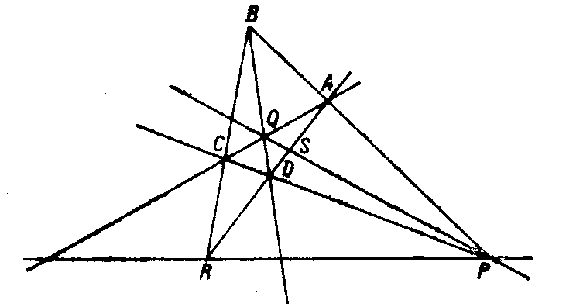Actions

A collection of four points \$A,B,C,D\$ (lying in a plane), no three of which lie on the same line, and the six lines connecting these points (cf. Fig.).Figure: q076010a

The points \$A,B,C,D\$ are called the vertices, and the lines \$AB,CD,AC,BD,BC,AD\$ are called the edges of the complete quadrangle. Edges that have no common vertex are called opposite; the points \$P,Q,R\$ of intersection of the opposite edges are called diagonal points.

If \$S\$ and \$T\$ are the points of intersection of the line \$PQ\$ with the lines \$AD\$ and \$BC\$, then the four points \$P,Q,S,T\$ form a harmonic quadruple of points. The dual figure to a quadrangle is called a quadrilateral — a collection of four lines (in a plane), no three of which contain a common point.